# probability distribution 2.pptx

30 May 2023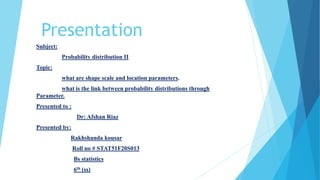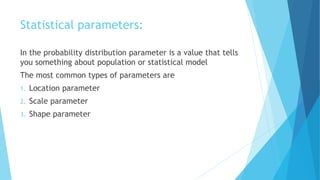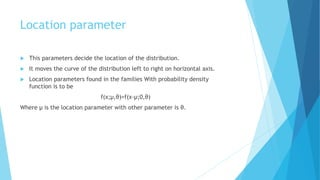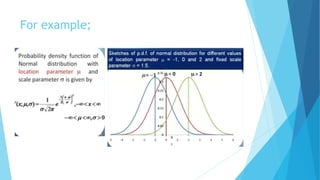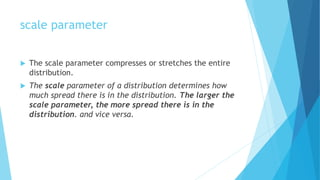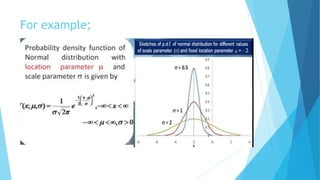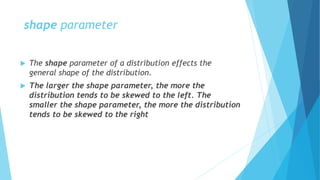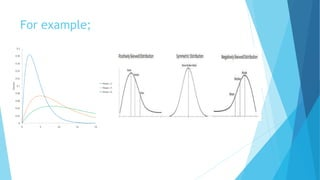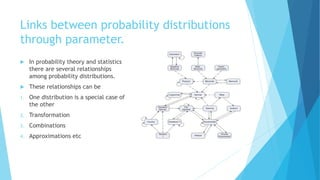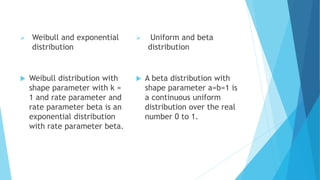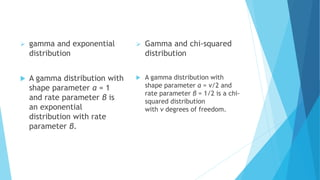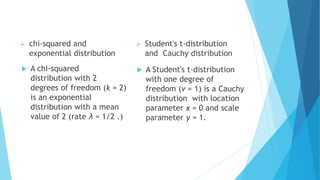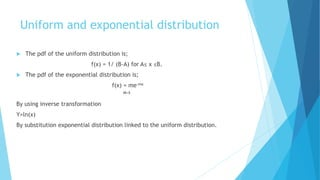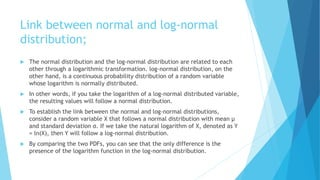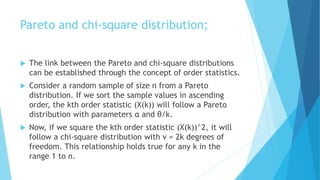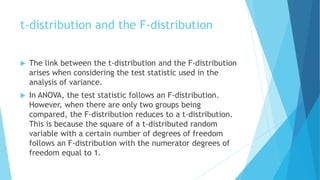1 sur 18

### probability distribution 2.pptx

• 2. Presentation Subject: Probability distribution II Topic: what are shape scale and location parameters. what is the link between probability distributions through Parameter. Presented to : Dr: Afshan Riaz Presented by: Rakhshanda kousar Roll no # STAT51F20S013 Bs statistics 6th (ss)
• 3. Statistical parameters: In the probability distribution parameter is a value that tells you something about population or statistical model The most common types of parameters are 1. Location parameter 2. Scale parameter 3. Shape parameter
• 4. Location parameter  This parameters decide the location of the distribution.  It moves the curve of the distribution left to right on horizontal axis.  Location parameters found in the families With probability density function is to be f(x;μ,θ)=f(x-μ;0,θ) Where μ is the location parameter with other parameter is θ.
• 6. scale parameter  The scale parameter compresses or stretches the entire distribution.  The scale parameter of a distribution determines how much spread there is in the distribution. The larger the scale parameter, the more spread there is in the distribution. and vice versa.
• 8. shape parameter  The shape parameter of a distribution effects the general shape of the distribution.  The larger the shape parameter, the more the distribution tends to be skewed to the left. The smaller the shape parameter, the more the distribution tends to be skewed to the right
• 10. Links between probability distributions through parameter.  In probability theory and statistics there are several relationships among probability distributions.  These relationships can be 1. One distribution is a special case of the other 2. Transformation 3. Combinations 4. Approximations etc
• 11.  Weibull and exponential distribution  Weibull distribution with shape parameter with k = 1 and rate parameter and rate parameter beta is an exponential distribution with rate parameter beta.  Uniform and beta distribution  A beta distribution with shape parameter a=b=1 is a continuous uniform distribution over the real number 0 to 1.
• 12.  gamma and exponential distribution  A gamma distribution with shape parameter α = 1 and rate parameter β is an exponential distribution with rate parameter β.  Gamma and chi-squared distribution  A gamma distribution with shape parameter α = v/2 and rate parameter β = 1/2 is a chi- squared distribution with ν degrees of freedom.
• 13.  chi-squared and exponential distribution  A Student's t-distribution with one degree of freedom (v = 1) is a Cauchy distribution with location parameter x = 0 and scale parameter γ = 1.  Student's t-distribution and Cauchy distribution  A chi-squared distribution with 2 degrees of freedom (k = 2) is an exponential distribution with a mean value of 2 (rate λ = 1/2 .)
• 14. Uniform and exponential distribution  The pdf of the uniform distribution is; f(x) = 1/ (B-A) for A≤ x ≤B.  The pdf of the exponential distribution is; f(x) = me-mx M=λ By using inverse transformation Y=ln(x) By substitution exponential distribution linked to the uniform distribution.
• 15. Link between normal and log-normal distribution;  The normal distribution and the log-normal distribution are related to each other through a logarithmic transformation. log-normal distribution, on the other hand, is a continuous probability distribution of a random variable whose logarithm is normally distributed.  In other words, if you take the logarithm of a log-normal distributed variable, the resulting values will follow a normal distribution.  To establish the link between the normal and log-normal distributions, consider a random variable X that follows a normal distribution with mean μ and standard deviation σ. If we take the natural logarithm of X, denoted as Y = ln(X), then Y will follow a log-normal distribution.  By comparing the two PDFs, you can see that the only difference is the presence of the logarithm function in the log-normal distribution.
• 16. Pareto and chi-square distribution;  The link between the Pareto and chi-square distributions can be established through the concept of order statistics.  Consider a random sample of size n from a Pareto distribution. If we sort the sample values in ascending order, the kth order statistic (X(k)) will follow a Pareto distribution with parameters α and θ/k.  Now, if we square the kth order statistic (X(k))^2, it will follow a chi-square distribution with ν = 2k degrees of freedom. This relationship holds true for any k in the range 1 to n.
• 17. t-distribution and the F-distribution  The link between the t-distribution and the F-distribution arises when considering the test statistic used in the analysis of variance.  In ANOVA, the test statistic follows an F-distribution. However, when there are only two groups being compared, the F-distribution reduces to a t-distribution. This is because the square of a t-distributed random variable with a certain number of degrees of freedom follows an F-distribution with the numerator degrees of freedom equal to 1.